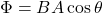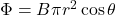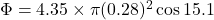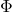## A uniform magnetic field passes through a horizontal circular wire loop at an angle 15.1 ° from the normal to the plane of the loop. The mag

Question

A uniform magnetic field passes through a horizontal circular wire loop at an angle 15.1 ° from the normal to the plane of the loop. The magnitude of the magnetic field is 4.35 T , and the radius of the wire loop is 0.280 m . Find the magnetic flux Φ through the loop.

in progress 0
5 months 2021-08-28T11:01:11+00:00 1 Answers 2 views 0

Magnetic flux through the loop is 1.03 T m²

Explanation:

Given:

Magnetic field, B = 4.35 T

Radius of the circular loop, r = 0.280 m

Angle between circular loop and magnetic field, θ = 15.1⁰

Magnetic flux is determine by the relation:….(1)

Here A represents area of the circular loop.

Area of circular loop, A = πr²

Hence, the equation (1) becomes:Substitute the suitable values in the above equation.= 1.03 T m²